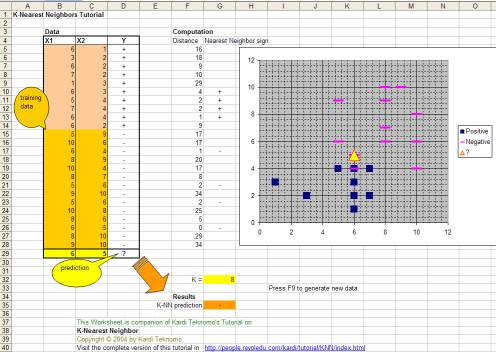## K Nearest Neighbor using Excel

The spreadsheet does not contain any macro. KNN algorithm use only simple MS excel functions

SMALL - return the k-th smallest value of the array input

COUNTIF - count number of cells that pass some simple criteria

RANDBETWEEN - to generate random integer between two values

You can change any value of K (number of neighbors) or the data to see the effect of prediction. Alternatively, you can just press F9 and it will automatically generate new data.

Yellow cells are for input, other color are results or computation.Preferable reference for this tutorial is

Teknomo, Kardi. K-Nearest Neighbors Tutorial. https:\\people.revoledu.com\kardi\tutorial\KNN\